Next: Cost Function Up: Nonlinear Independent Component Analysis Previous: Introduction

# Model Structure

The nonlinear mapping f is modelled by a multi-layer perceptron (MLP) network having two layers.

 f(s(t)) = B g(A s(t) + a) + b (2)

The activation function for each of the nonlinear hidden neurons is the hyperbolic tangent, that is,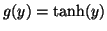. In addition to the weight matrices A and B, both the hidden neurons and the linear output neurons have biases, denoted by a and b, respectively.

In order to apply the Bayesian approach, each unknown variable in the network is assigned a probability density function (pdf). We apply the usual hierarchical definition of priors. For many parameters, for instance the biases a, it is difficult to assign a prior distribution but we can utilise the fact that each bias occurs in a similar role in the network by assuming that the distribution for each element of vector a has the same, albeit unknown distribution which is then modelled by a parametric distribution. These new parameters need to be assigned a prior also, but there are far fewer of them.

The noise n(t) is assumed to be independent and Gaussian with a zero mean. The variance can be different on different channels, and hence the algorithm can be more accurately be called nonlinear independent factor analysis. Given s(t), the variance of x(t) is due to the noise. Therefore x(t) has the same distribution as the noise except with the mean f(s(t)).

The distribution of each of the sources is modelled by a mixture of Gaussians. We can think that for each source si(t) there is a discrete process which produces a sequence Mi(t) of indices which tell from which Gaussian each si(t) is originated. Each Gaussian has its own mean and variance and the probability of different indices is modelled by a soft-max distribution.

The model is defined by the following set of distributions:

 x(t)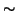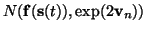(3) P(Mi(t) = l) =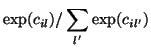(4) si(t)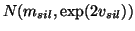(5) AN(0, 1) (6) B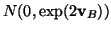(7) a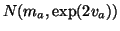(8) b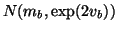(9) vn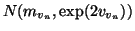(10) c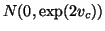(11) ms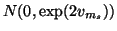(12) vs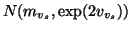(13) vB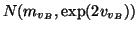(14)

The prior distributions of ma, va, mb, vb and the eight hyperparameters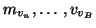are assumed to be Gaussian with zero mean and standard deviation 100, that is, the priors are assumed to be very flat.

The parametrisation of all the distributions is chosen such that the resulting parameters have a roughly Gaussian posterior distribution. This is because the posterior will be modelled by a Gaussian distribution. For example, the variance of the Gaussian distributions is parametrised on a logarithmic scale.

Model indeterminacies are handled by restricting some of the distributions. There is a scaling indeterminacy between the matrix A and the sources, for instance. This is taken care of by setting the variance of A to unity instead of parametrising and estimating it. For the second layer matrix B there is no such indeterminacy. The variance of each column of the matrix is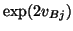. The network can effectively prune out some of hidden neurons by setting the outgoing weights of the hidden neurons to zero, and this is easier if the variance of the corresponding columns of B can be given small values.Next: Cost Function Up: Nonlinear Independent Component Analysis Previous: Introduction
Harri Lappalainen
2000-03-03